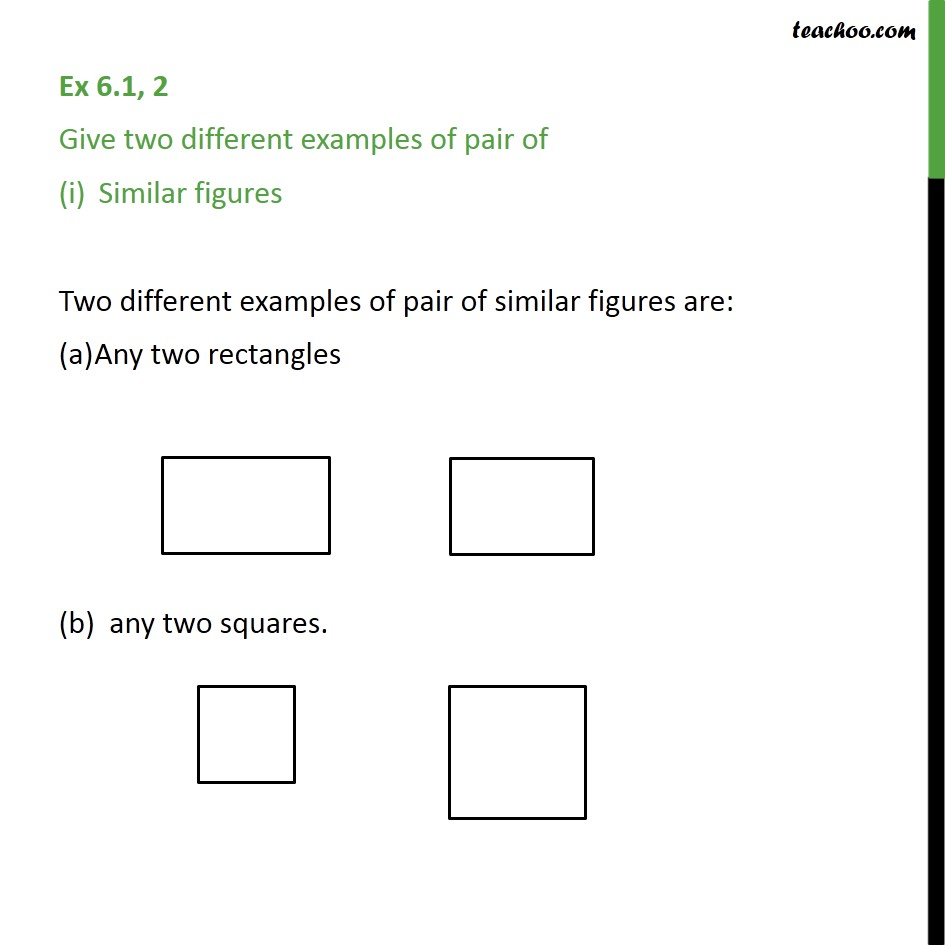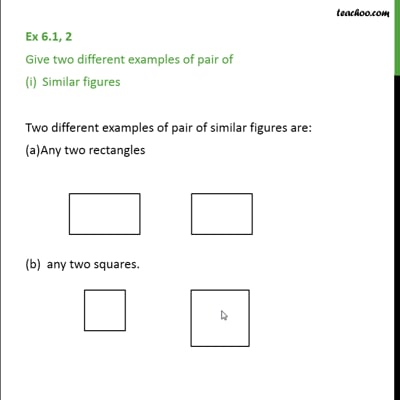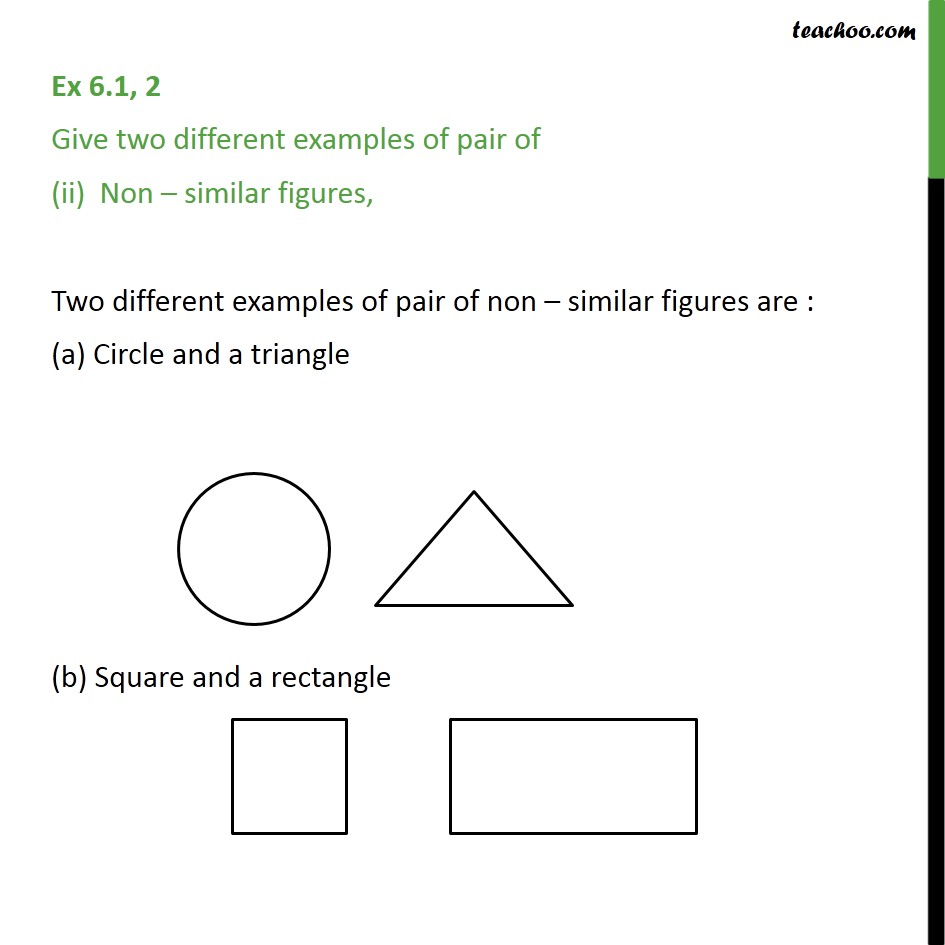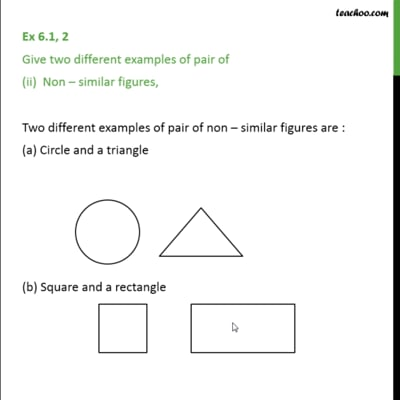Ex 6.1

Chapter 6 Class 10 Triangles (Term 1)
Serial order wiseThis video is only available for Teachoo black usersThis video is only available for Teachoo black users

### Transcript

Ex 6.1, 2 Give two different examples of pair of Similar figures Two different examples of pair of similar figures are: Any two rectangles (b) any two squares. Ex 6.1, 2 Give two different examples of pair of (ii) Non similar figures, Two different examples of pair of non similar figures are : (a) Circle and a triangle (b) Square and a rectangle